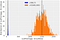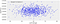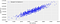# The Bayesian Housing Price for competitions is finally here — Part 1

## The imagination of a world where we finally understand the difference between frequentist and bayesian statistics.

ver since I started to dedicate time to understand some of the intricate details of A.I, I have been thinking: “Ok, some of the exercises I see on the Internet, I would try to turn it into Bayesian inference for practice.” In time, I realized that to proceed in that direction; I needed two essential elements: (1) an understanding of the difference between frequentist and Bayesian statistics, and (2) a friendly computational tool that allowed me to accomplish that goal without quitting all my other social duties. With dedication and effort, I am on my way to fulfill (1) “not perfectly of course but better than before”, and… I did find the (2). PyMC3 assisted me in transforming the classical “Housing Price” exercise into Bayesian. If you want to convert some of your classical results into Bayesian notebooks, stay around because I will try to give some tips to do that.

Now, because I’d like to start very very basic, I won’t actually download the Housing Price data. That will come later in Part 2 of this serie, but I will create two, or three features, synthetically and then explain how we will use them for the “real data” in the next article.

# Generating synthetic data:

First, let’s create two very basic features for our Bayesian Machine Learning problem. We are choosing two numerical features: LotFrontage and GrLivArea. In the future, we will add some synthetic categorical features as well. You might find inspiration by looking at this notebook.

Let’s put hands-on:

# Importing basic packagesimport numpy as npimport pandas as pdimport warningsimport arviz as azimport matplotlib.pyplot as pltimport pymc3 as pmwarnings.simplefilter(action=”ignore”, category=FutureWarning)### For pretty plots.%config InlineBackend.figure_format = ‘retina’az.style.use(“arviz-darkgrid”)print(f”Running on ArviZ v{az.__version__}”)

Now, the distribution of our data could be any of the famous or popular distributions: Poisson, Bernoulli, Binomial, etc, but here we assume the two features are Normally distributed:

# Only knowledge of what a Gaussian is... is needed.# Size of our datasetsize = 1000# creating synthetic features … (#1) LotFrontagemean1=60sd1=7## creating a normal distribution dataX1 = np.random.normal(mean1, sd1, size) # x ~ N(60,7)# creating synthetic features … (#2) GrLivAreamean2=1500sd2=200## creating a normal distribution dataX2 = np.random.normal(mean2, sd2, size) # x ~ N(mu,sd)## We might add more features here ...# ... (#3)# ... (#4)######## ## plotting histographsplt.hist(X1, 100, label=’$\mathcal{N}$(60,7)’)plt.hist(X2, 100, label=’$\mathcal{N}$(1500,200)’)## plotting mean linesplt.axvline(X1.mean(), color=’k’, linestyle=’dashed’, linewidth=2)plt.axvline(X2.mean(), color=’k’, linestyle=’dashed’, linewidth=2)## pretty legends:plt.legend()## showing the graphplt.show()Two normally distributed synthetically created features for our Bayesian ML Housing problem.

# The model

Here we have to imagine what the relation between our target variable (Y=SalePrice) and the two features is going to be. We assume the relationship is linear between these three variables:

# True parameter valuesalpha, sigma= 1, 10000beta = [25, 120]# Simulate outcome variableY = alpha + beta * X1 + beta * X2 + np.random.randn(size) * sigma

It is good practice to visualize what we do. Let’s see how the target dependence with the two features is:We can easily see a weak dependence of the SalePrice with the LotFrontage.We can easily see a strong dependence of the SalePrice with the GrLivArea.

# Bayesian modeling and PyMC3:

From the classical point of view, the formulation of the problem is like this:

Given a set of data, target vs features, we want to propose a model, followed by the finding of the parameters that best represent that model so we can do predictions with it.

In summary all you care about is related with the computation of the likelihood:

which literally reads: “the probability of the data given (|) the parameters”. Alternatively:

written in this way, (2) and (3) express compactly the difference between the two schools of statistics. I’d like to add:

In Bayes’ view, we transform the parameters into random variables.

It is worth noticing that the PyMC3 developer team made an effort to deliver sintaxis for the construction of a model as close as posible to equation (1):

with pm.Model() as model:     α = pm.Normal("α", mu=0.8, sigma=0.75)    β1 = pm.Normal("β1", mu=25, sigma=5)    β2 = pm.Normal("β2", mu=120, sigma=5)    σ = pm.HalfNormal("σ", sigma=5000)        𝜇 = α + β1*X1 + β2*X2     Likelihood = pm.Normal(‘Likelihood’, mu=𝜇, sigma=σ, observed=Y)

Once our model is created, we generate the data that allows us to sampling and produce posteriors for our parameters alpha, beta1 and beta2. Wait a moment, what is a posterior then? It is not my intention to dive deep into the theory of Bayesian statistics, but to show you that they are two different manners of doing inferences. While frequentists care about the likelihood of the Data given the parameters of a model, in Bayes we sigh for the computation of the Parameters given de Data, nearly mirroring the former.

with model: trace = pm.sample(3000)

which is visually represented:

with model: az.plot_trace(trace, compact=False, combined=False)

Let’s clarify some of the properties in the plots above:

• The left represent posteriors, the right represent the 3000 runs for the sampling.
• There are two chains: one in orange and the other in blue-solid lines.
• The “β1 and β2 plots” are the two coefficients multiplying the features in equation (1).

From posteriors we inference best parameters, allowing us to construct predictions for values that are not in the initial set of data.

We see the similarities between these estimations and the values we used to create Y (SalePrice).

We trained a Bayesian model for the Housing Price.

# Conclusion

The Bayesian framework provide us of a powerful methodology to produce Machine Learning (ML) flows for training linear models as is demonstrated here. Feel free to play around with the creation of new features, variation of the synthetic parameters, and simulations for samplings. A special feature of equation (3) is that any prior information we have about the parameters can be easily incorporated. PyMC3 is a very intuitive tool that allows us to performance this Bayesian ML training. In the next article of this serie we will create categorical features and try to download the real data of the Housing Price competition.

Thanks for reading. Let me know if you have any feedback.

Physicist and Data Scientist.

## More from Jose Manuel Ramirez

Physicist and Data Scientist.